关于一个无穷小数证明题 热

TestXd 发布于 2011/09/11 20:14

年薪达不到25W大数据工程师、拿不到Offer全额退款->>>《编程之美》的2.6节也和这个一样，证明利用了相同的思想，明明是错的

1=1=（1/3）*3=0.999999……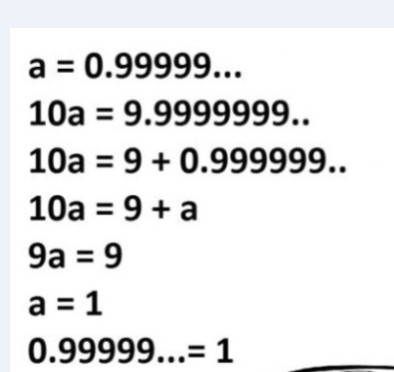以下是问题补充：

@TestXd：谢谢各位了，看大家的争论也是一种享受啊 (2011/09/15 22:59)

1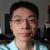引用来自“xyz555”的答案

0.99999..... == 1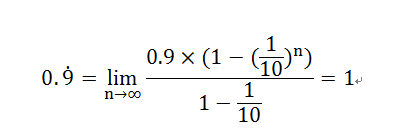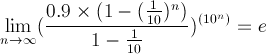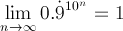a = 0.9
10a != 9 + 0.9

a = 0.99
10a != 9 + 0.99

a = 0.999
10a != 9 + 0.999

a = 0.999...
10a != 9 + a
a != 11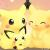引用来自“ChenQi”的答案

"10a = 9 + a"

"10a = 9a + a"吧？

除非 a=1 这个 "10a = 9 + a" 才成立

0.9+0.09+0.009+... = a
10a = 9 + 0.9 + 0.09 + ... = 9 + a

“0.9+0.09+0.009+... = a

10a = 9 + 0.9 + 0.09 + ... = 9 + a ”

10a = 9 + 0.9 + 0.09 + ... 这个怎么成立？要知道现在你并不知道 0.9+0.09+0.009+... = 1，这是需要证明的。a 只是你假设的，a到底等于多少是不知道的，如果a = 2你的等式能成立吗？这样你不能断言 10a = 9 + a

0.9+0.09+0.009+... = a

10a = 9 + 0.9 + 0.09 + ... = 9 + a

0.1 + 0.01 + 0.001 + ..... =  a

10a = 9 +0.1 + 0.01 + 0.001 + ..... = 9 + a

10 + 100 + 1000 + 10000 + ...... =  a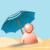10a = 1 + a, a = 1/9 你把a = 1/9代回去等式相等吗？等式变换时要做等价变换，10a = 9a + 1也不对。因为你在这里已经限定了a = 1等式才成立，那还要证明什么。0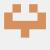引用来自“Jeky”的答案

0.9的的循环就等于1啊....没错啊

0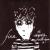引用来自“Jeky”的答案

0.9的的循环就等于1啊....没错啊

1/3 = 0.3的循环 两边同乘3  1=0.9的循环

1/9 = 0.1的循环 两边同乘9  1=0.9的循环

0.9的循环可以看成以0.9为首项，以0.1为公比的等比数列的和：0
i

00.99999..... == 10
0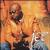0.9... = 0.3... + 0.6...

0.9... = 1/3 + 2/3

0.9... = 1

0